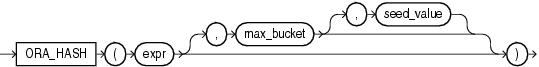## ORA_HASH

SyntaxDescription of the illustration ora_hash.eps

Purpose

`ORA_HASH` is a function that computes a hash value for a given expression. This function is useful for operations such as analyzing a subset of data and generating a random sample.

• The `expr` argument determines the data for which you want Oracle Database to compute a hash value. There are no restrictions on the length of data represented by `expr`, which commonly resolves to a column name. The `expr` cannot be a `LONG` or LOB type. It cannot be a user-defined object type unless it is a nested table type. The hash value for nested table types does not depend on the order of elements in the collection. All other data types are supported for `expr`.

• The optional `max_bucket` argument determines the maximum bucket value returned by the hash function. You can specify any value between 0 and 4294967295. The default is 4294967295.

• The optional `seed_value` argument enables Oracle to produce many different results for the same set of data. Oracle applies the hash function to the combination of `expr` and `seed_value`. You can specify any value between 0 and 4294967295. The default is 0.

The function returns a `NUMBER` value.

Examples

The following example creates a hash value for each combination of customer ID and product ID in the `sh.sales` table, divides the hash values into a maximum of 100 buckets, and returns the sum of the `amount_sold` values in the first bucket (bucket 0). The third argument (5) provides a seed value for the hash function. You can obtain different hash results for the same query by changing the seed value.

```SELECT SUM(amount_sold)
FROM sales
WHERE ORA_HASH(CONCAT(cust_id, prod_id), 99, 5) = 0;

SUM(AMOUNT_SOLD)
----------------
989431.14```